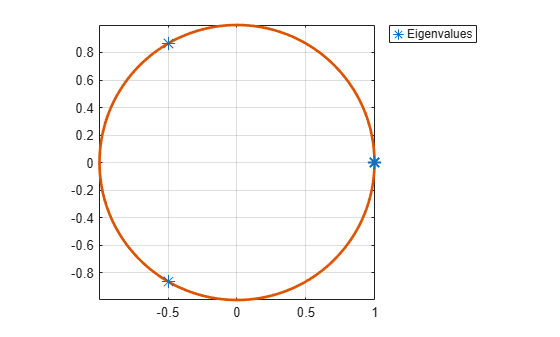# isergodic

Check Markov chain for ergodicity

## Syntax

``tf = isergodic(mc)``

## Description

example

``tf = isergodic(mc)` returns `true` if the discrete-time Markov chain `mc` is ergodic and `false` otherwise.`

## Examples

collapse all

Consider this three-state transition matrix.

`$P=\left[\begin{array}{ccc}0& 1& 0\\ 0& 0& 1\\ 1& 0& 0\end{array}\right].$`

Create the Markov chain that is characterized by the transition matrix P.

```P = [0 1 0; 0 0 1; 1 0 0]; mc = dtmc(P);```

Determine whether the Markov chain is ergodic.

`isergodic(mc)`
```ans = logical 0 ```

`0` indicates that the Markov chain is not ergodic.

Visually confirm that the Markov chain is not ergodic by plotting its eigenvalues on the complex plane.

```figure; eigplot(mc);```All three eigenvalues have modulus one. This result indicates that the period of the Markov chain is three. Periodic Markov chains are not ergodic.

## Input Arguments

collapse all

Discrete-time Markov chain with `NumStates` states and transition matrix `P`, specified as a `dtmc` object. `P` must be fully specified (no `NaN` entries).

## Output Arguments

collapse all

Ergodicity flag, returned as `true` if `mc` is an ergodic Markov chain and `false` otherwise.

collapse all

### Ergodic Chain

A Markov chain is ergodic if it is both irreducible and aperiodic. This condition is equivalent to the transition matrix being a primitive nonnegative matrix.

## Algorithms

• By Wielandt's theorem , the Markov chain `mc` is ergodic if and only if all elements of Pm are positive for m = (n – 1)2 + 1. P is the transition matrix (`mc.P`) and n is the number of states (`mc.NumStates`). To determine ergodicity, `isergodic` computes Pm.

• By the Perron-Frobenius Theorem , ergodic Markov chains have unique limiting distributions. That is, they have unique stationary distributions to which every initial distribution converges. Ergodic unichains, which consist of a single ergodic class plus transient classes, also have unique limiting distributions (with zero probability mass in the transient classes).

 Gallager, R.G. Stochastic Processes: Theory for Applications. Cambridge, UK: Cambridge University Press, 2013.

 Horn, R., and C. R. Johnson. Matrix Analysis. Cambridge, UK: Cambridge University Press, 1985.

 Wielandt, H. "Unzerlegbare, Nicht Negativen Matrizen." Mathematische Zeitschrift. Vol. 52, 1950, pp. 642–648.